Constructing angles using protractor

Practical Geometry
Serial order wise

Here, we will draw right angle i.e. 90° using protractor.

To draw 90° using compass, please check Ex 11.1, 1 of Class 9 NCERT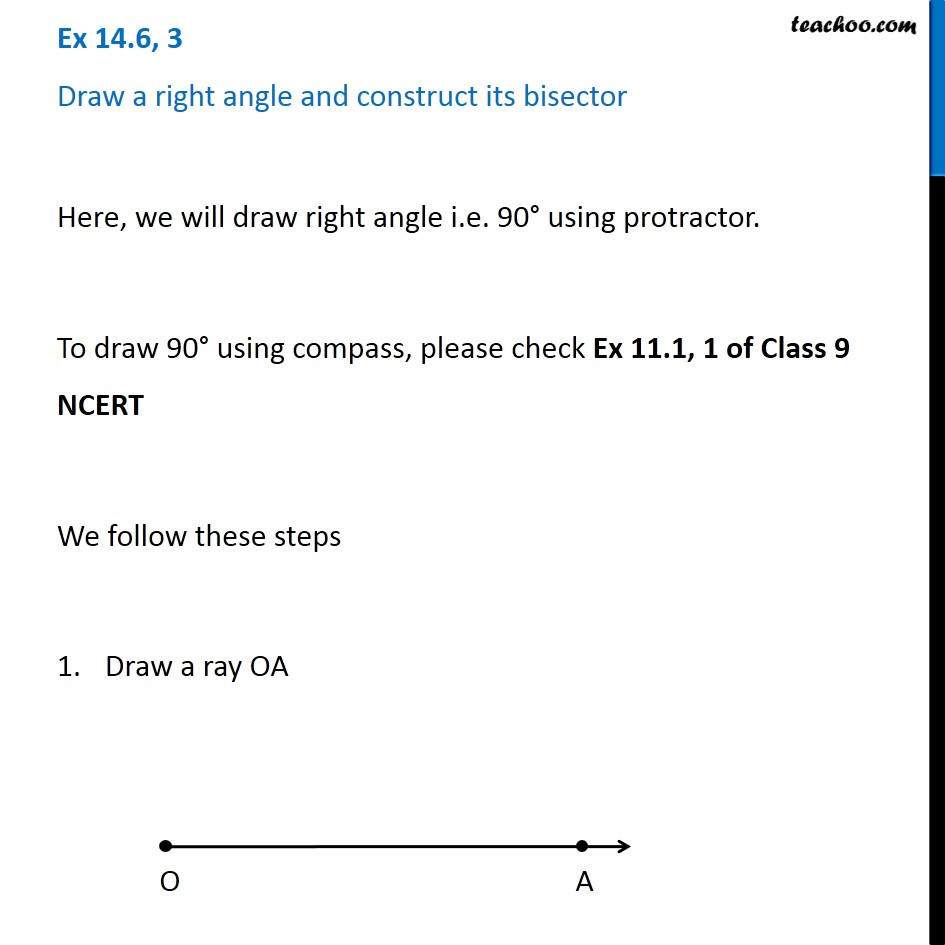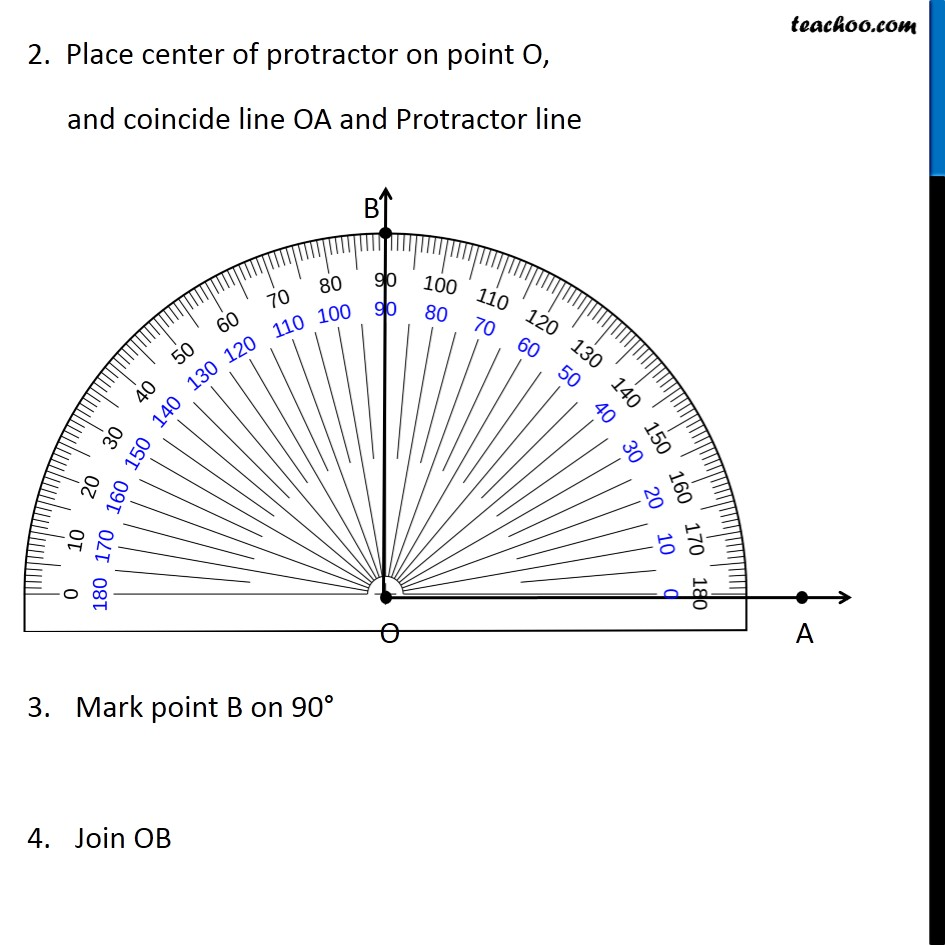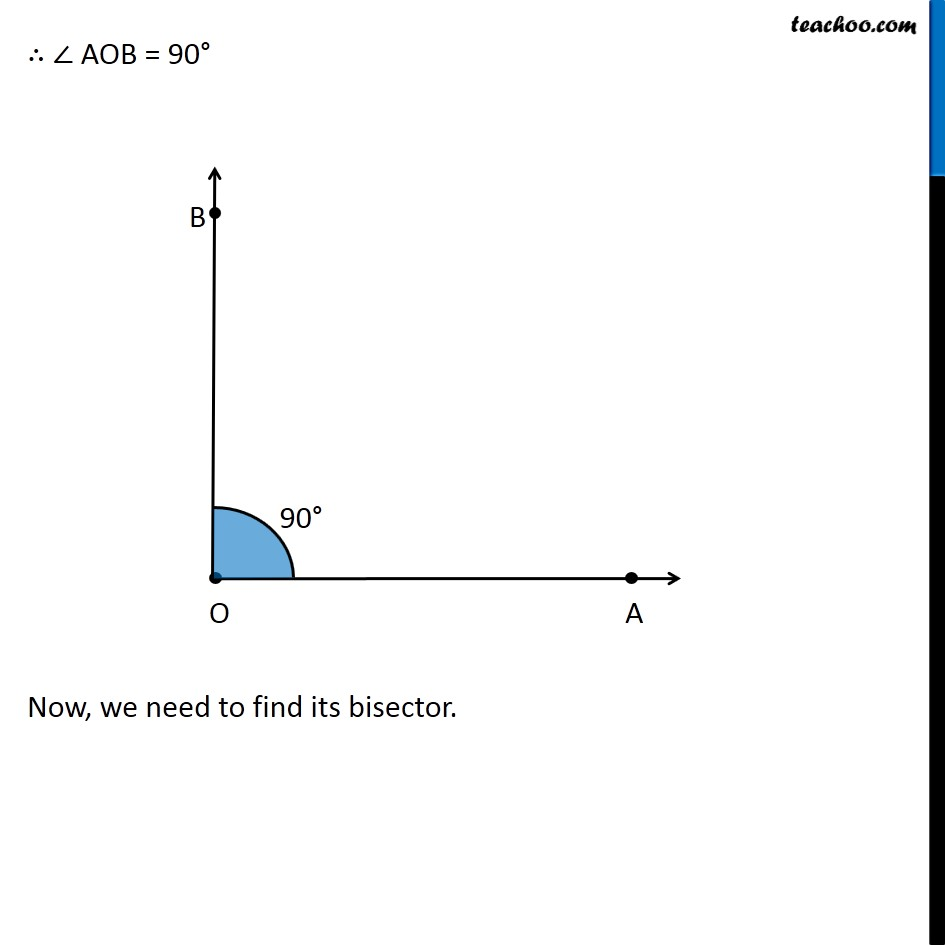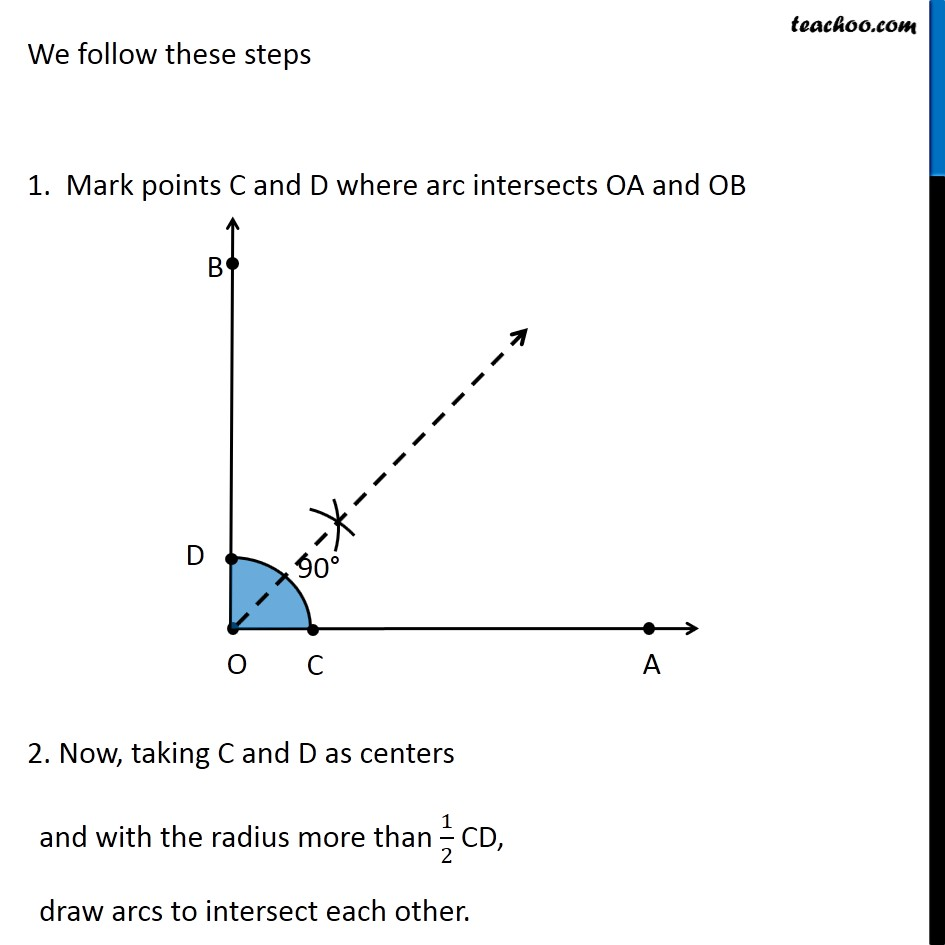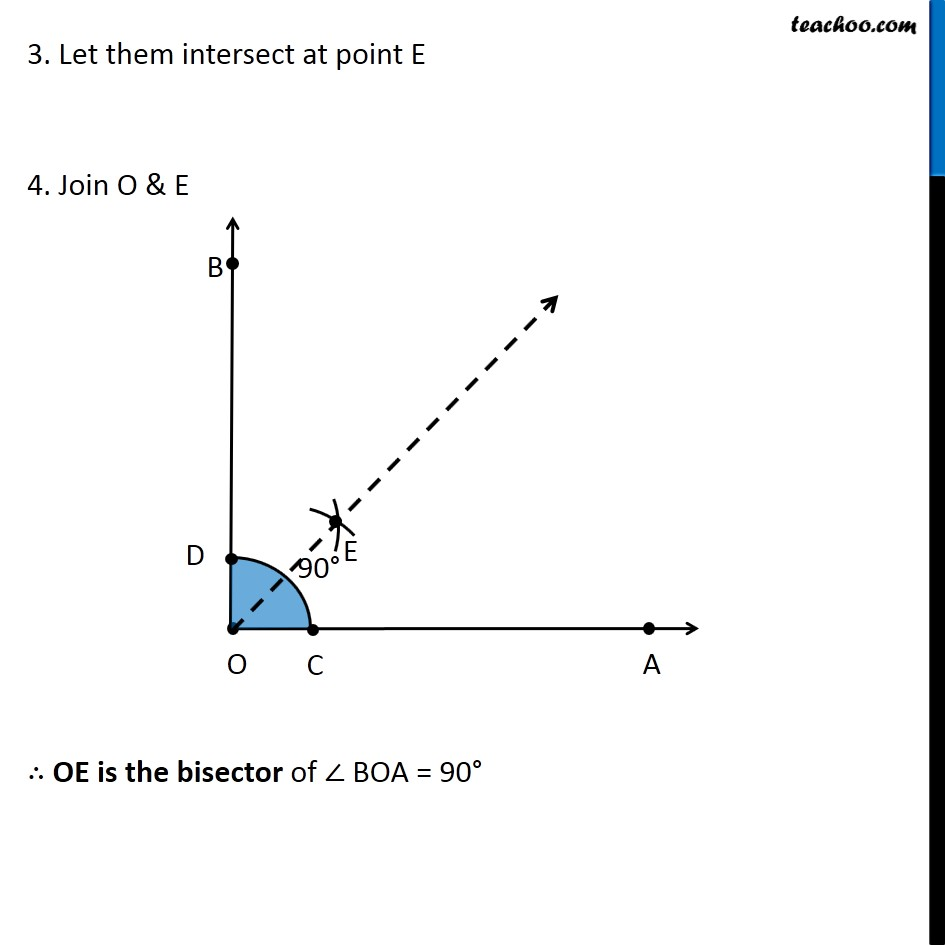Learn in your speed, with individual attention - Teachoo Maths 1-on-1 Class

### Transcript

Question 3 Draw a right angle and construct its bisector Here, we will draw right angle i.e. 90° using protractor. To draw 90° using compass, please check Ex 11.1, 1 of Class 9 NCERT We follow these steps Draw a ray OA 2. Place center of protractor on point O, and coincide line OA and Protractor line Mark point B on 90° Join OB ∴ ∠ AOB = 90° Now, we need to find its bisector. We follow these steps 1. Mark points C and D where arc intersects OA and OB 2. Now, taking C and D as centers and with the radius more than 1/2 CD, draw arcs to intersect each other. 3. Let them intersect at point E 4. Join O & E ∴ OE is the bisector of ∠ BOA = 90°